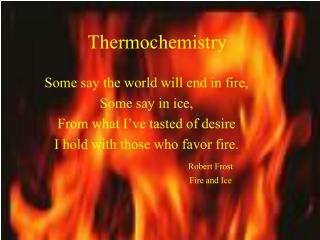DownloadDownload PresentationThermochemistry

# Thermochemistry

Download Presentation## Thermochemistry

- - - - - - - - - - - - - - - - - - - - - - - - - - - E N D - - - - - - - - - - - - - - - - - - - - - - - - - - -
##### Presentation Transcript

1. Thermochemistry Some say the world will end in fire, Some say in ice, From what I’ve tasted of desire I hold with those who favor fire. Robert Frost Fire and Ice

2. Principles of Heat Flow • Thermochemistry is the study of heat flow • System: the part of the universe on which we are focused • Surroundings: exchange energy with system (in close contact with system)

3. Composition Temperature Pressure (remind you of stp?) Heat flow (q) q is + when heat flows into the system from the surroundings (heating of a cup of coffee) q is - when heat flows out of the system into the surrounds (cooling of a cup of coffee) State Properties if X is a state property, then ∆X = Xfinal-Xinitial Heat Flow is NOT a state property

4. Endo- and Exothermic Processes • An endothermic process (q>0) in which heat flows form the surroundings into the reaction system. • And exothermic process(q<0) in which heat flows from the reaction system into the surroundings surroundings system OUT exothermic IN endothermic

5. q = C x ∆t ∆t = tfinal - tinitial For a pure substance q = m x c x ∆t m is mass c is specific heat Specific heat is the amount of energy necessary to raise the temperature of 1 gram of a substance 1 degree C. Magnitude of Heat Flow t is temperature q is heat flow C is heat capacity q is measured in joules

6. Example • How much heat is needed to raise the temperature of a 3000 gallon pool from 10 C to 20 C? The specific heat of water is 4.18 J/goC 1. Q = m x c x ∆t 2. Plug and chug

7. Calorimetry • Calorimeter: device that measures the heat flow in a reaction • qreaction = -q calorimeter • qreaction = -Ccal x ∆t • “old” heat unit is the calorie • 1 cal = 4.184 J (c) • 1 kcal = 4.184 kJ (C)

8. Coffee Cup Calorimeter • Heat from the reaction inside the innermost cup flows out to the water in the outer cup. • Ccal = mwater x cwater • qreaction =-mwater x cwater x ∆t

9. Bomb Calorimetry

10. The reaction between H and Cl : H2(g) + Cl2(g) --> 2HCl(g) can be studied in a bomb calorimeter. It is found that when a 1.00 g sample of H2 reacts completely the temperature rises from 20.00 C to 29.82 C. Taking the heat capacity of the calorimeter to be 9.33 kj?oC, calculate the amount of heat evolved in the reaction. Which equation to use? Example qreaction = -Ccal x ∆t Plug and chug Answer: -91.6 kJ exothermic

11. When 1.00 g of CaCl2 is added to 50.0 g of water in a coffee-cup calorimeter, it dissolves CaCl2(s) --> Ca2+(aq) + 2Cl-(aq) And the temperature rises from 25.00 C to 28.51 C. Assuming that all the heat given off by the reaction is transferred to the water, calculate q for the reaction system. Which equation? Example qreaction = -mwater x cwater Plug and chug Answer: -734 J • exothermic

12. Enthalpy (H) • Enthalpy is a type of chemical energy (heat content) • Under constant P, heat flow for a reaction system is equal to the difference in enthalpy between products and reactants qreaction= ∆H= Hproducts- Hreactants

13. Enthalpy continued • For an exothermic reaction q = ∆H< 0 • For an endothermic reaction q = ∆H> 0 The enthalpy of a substance, like its volume, is a state property

14. A chemical equation which shows the enthalpy relation between products and reactants NH4NO3(s) --> NH4+(aq)+ NO3-(aq) ∆H = +28.1 kJ The sign of ∆H indicates endothermic (+) or exothermic (-) Thermochemical Equations The coefficients represent numbers of moles The phases must be specified--significant differences at different phases The value quoted for H applies when products and reactants are at the same temp (usually 25C) H2(g)+ Cl2(g) --> 2HCl ∆H = -185kJ

15. The magnitude of ∆H is directly proportional to the amount of reactant or product The ∆H for a reaction is equal in magnitude but opposite in sign to ∆H for the reverse reaction. Rules of Thermochemistry H2(g)+ Cl2(g) --> 2HCl ∆H = -185kJ H2(g)+ Cl2(g) --> 2HCl(g) ∆H = -185 kJ -185 kJ1 mol H2 1 mol Cl2 -185 kJ -185 kJ 2 mol HCl 2HCl (g)--> H2 (g) + Cl2 (g) ∆H = 185 kJ Heat of fusion : heat absorbed when a solid melts Heat of vaporization: heat absorbed when a liquid vaporizes

16. Example

17. Enthalpy of Formation

18. Example

19. Example